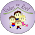## Elementary Studies

### Division Facts

Division is one of the four basic operations of arithmetic, the other being addition, subtraction, and multiplication. Once kids are fluent in multiplication facts, teaching division becomes easy. My today’s post is on introducing the concept of division to the young learners.

#### Introducing Division

Division is a sharing operation, where objects are shared equally into a number of groups.

Hands on Activity: Give 9 pennies or counters and 3 bowls to each child and tell them to put equal pennies/counters in each bowl.

If the result is as shown in picture, tell them - This is what the division is.

#### Multiplication and Division relationship (Multiplication and Division Fact Family)

Multiplication and Division has inverse relationship just like addition and subtraction.

Example:
Multiplication Equation:  4 x 5 = 20
The two division equations will be:
20 ÷ 5 = 4
20 ÷ 4 = 5

Similarly, we can derive multiplication equations from division equation.
35 ÷ 5 = 7

The two multiplication equations will be:
7 x 5 = 35
5 x 7 = 35

Understanding the multiplication and division relationship is very important in solving the division problems.

#### Division Vocabulary

Dividend: A number to be divided by another number.
Divisor: A number by which another number is divided.
Quotient: The result of division.

This division vocabulary poster is also included in my division packet.

You can use this division booklet to teach division facts up to 12 without a remainder. Worksheets covering the above concepts are included in this packet.

This division packet can be purchased from HERE

Happy Teaching!

Shimps

#### 1 comment:

1.I love the coins in the cups idea for division.